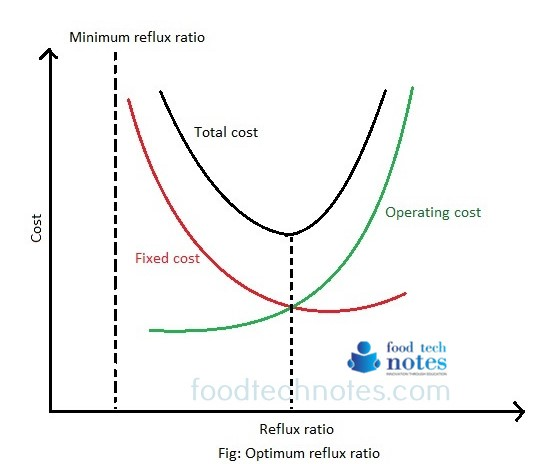# Optimum Reflux Ratio

For actual design of distillation, we use neither minimum neither infinite reflux ratio. Nonetheless we use these two numbers to identify lower and upper limit of usable reflux ratio. Intuitively the reflux ratio to be used should fall in between minimum and infinite reflux ratio.For the case of total reflux, number of plate is minimum but lower diameter is infinite. This corresponds to an infinite cost of tower as well as steam and cooling water. Also for minimum reflux, the number of trays is infinite which again gives infinite cost. We must therefore evaluate tradeoff between reflux ratio ‘R’ and number of theoretical trays ‘N’. To select proper value of ‘R’ requires complete economic balance on fixed cost of tower and operating costs.

For new design, the reflux ratio to be used should be optimum or the most economical for which total cost will be least. There are two components of total cost

1. Fixed cost (Purchase of distillation column, reboiler, condenser and other equipment)
2. Operating cost (cost associated with operation of plant ex; pump horsepower, cooling water, steam)

At minimum reflux ratio, the fixed cost is infinite (due to no. of infinite stages required) but the operating cost is minimum because minimum amount of liquid is to be handled (ex; consuming lesser pump horsepower, lesser cooling capacity, lesser heating capacity).

As reflux ratio increases, less stages are required but larger equipment are needed to handle increased refluxed liquid and re-boiled vapor load. Thus the fixed cost initially decreased but eventually increase again when the reflux ratio approaches total reflux. Here the fixed cost fall through minimum and then rise again to infinity. As for the operating cost, it will continue to increase with increasing reflux ratio. The total cost which is the sum of fixed cost and operating cost must therefore pass through a minimum. The reflux ratio at this minimum total cost is the optimum (economical) reflux ratio. Typically the optimum reflux ratio is 1.2 to 1.5 times Rmin.